Anzeige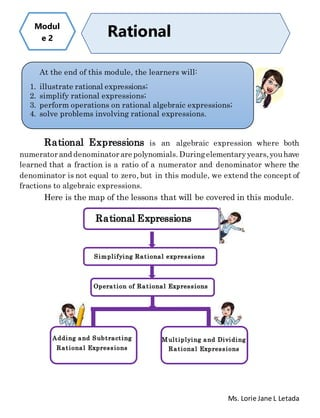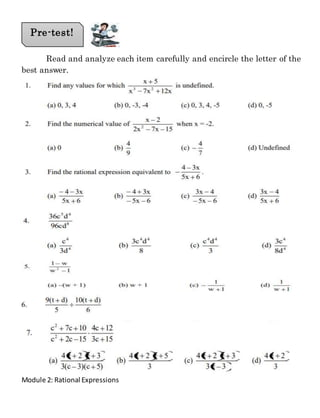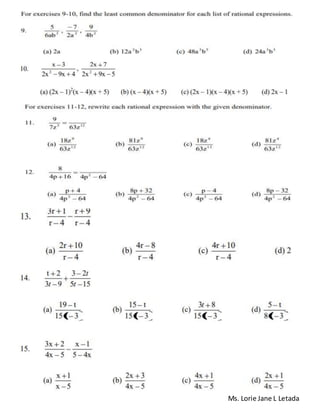Anzeige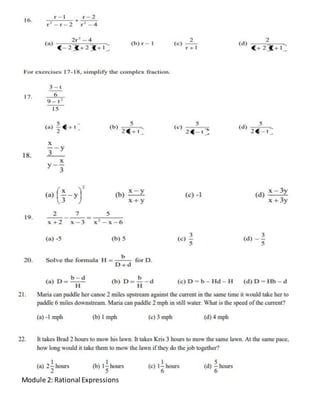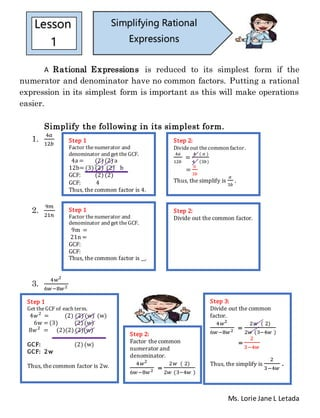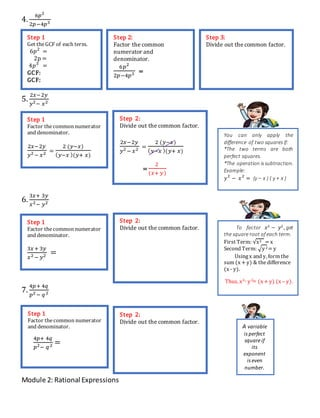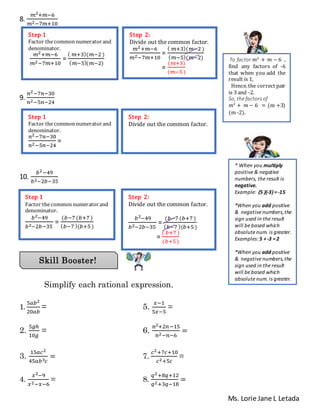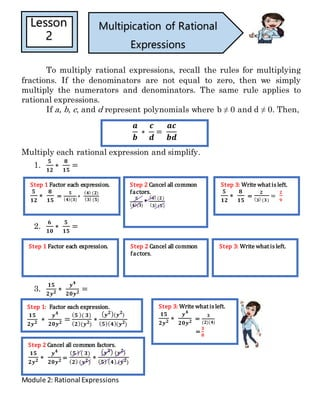Anzeige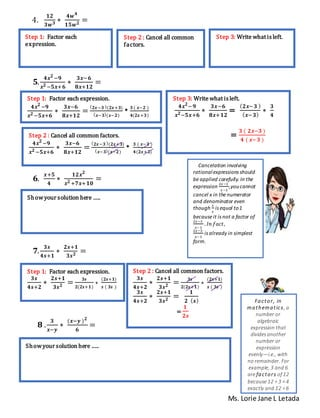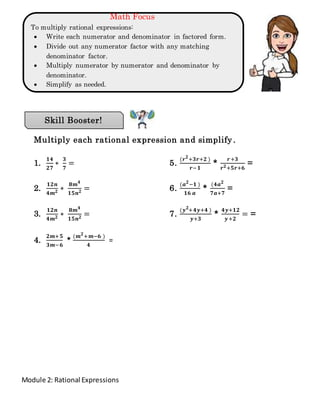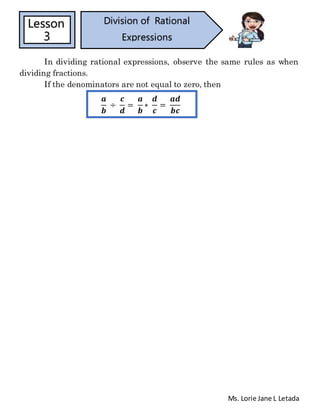Nächste SlideShareRational Expressions Module
1 von 12
Anzeige

### Rational Expressions

1. Ms. Lorie Jane L Letada Rational Expressions is an algebraic expression where both numerator and denominator are polynomials. Duringelementaryyears,youhave learned that a fraction is a ratio of a numerator and denominator where the denominator is not equal to zero, but in this module, we extend the concept of fractions to algebraic expressions. Here is the map of the lessons that will be covered in this module. Modul e 2 Rational Expressions At the end of this module, the learners will: 1. illustrate rational expressions; 2. simplify rational expressions; 3. perform operations on rational algebraic expressions; 4. solve problems involving rational expressions. Rational Expressions Simplifying Rational expressions Operation of Rational Expressions Adding and Subtracting Rational Expressions Multiplying and Dividing Rational Expressions
2. Module 2: Rational Expressions Read and analyze each item carefully and encircle the letter of the best answer. Pre-test!
3. Ms. Lorie Jane L Letada
4. Module 2: Rational Expressions
5. Ms. Lorie Jane L Letada A Rational Expressions is reduced to its simplest form if the numerator and denominator have no common factors. Putting a rational expression in its simplest form is important as this will make operations easier. Simplify the following in its simplest form. 1. 4𝑎 12𝑏 2. 9𝑚 21𝑛 3. 4𝑤2 6𝑤−8𝑤2 Lesson 1 Simplifying Rational Expressions Step 1 Factor thenumerator and denominator and get theGCF. 4a = (2) (2) a 12b= (3) (2) (2) b GCF: (2) (2) GCF: 4 Thus, the common factor is 4. Step 2: Divideout thecommon factor. 4𝑎 12𝑏 = 4 ( 𝑎 ) 4 (3𝑏) = 𝑎 3𝑏 Thus, the simplify is 𝑎 3𝑏 . Step 1 Factor thenumerator and denominator and get the GCF. 9m = 21n = GCF: GCF: Thus, the common factor is __. Step 2: Divide out the common factor. Step 1 Get theGCF of each term. 4𝑤2 = (2) (2) (w) (w) 6w = (3) (2) (w) 8𝑤2 = (2)(2) (2)(w) GCF: (2) (w) GCF: 2w Thus, the common factor is 2w. Step 2: Factor the common numerator and denominator. 4𝑤2 6𝑤−8𝑤2 = 2𝑤 ( 2) 2𝑤 (3−4𝑤 ) Step 3: Divide out the common factor. 4𝑤2 6𝑤−8𝑤2 = 2𝑤 ( 2) 2𝑤 (3−4𝑤 ) = 2 3−4𝑤 Thus, the simplify is 2 3−4𝑤 .
6. Module 2: Rational Expressions 4. 6𝑝2 2𝑝−4𝑝3 5. 2𝑥−2𝑦 𝑦2− 𝑥2 6. 3𝑥+ 3𝑦 𝑥2− 𝑦2 7. 4𝑝+ 4𝑞 𝑝2 − 𝑞2 Step 1 Get theGCF of each term. 6𝑝2 = 2p = 4𝑝3 = GCF: GCF: Step 2: Factor the common numerator and denominator. 6𝑝2 2𝑝−4𝑝3 = Step 3: Divide out the common factor. Step 1 Factor thecommon numerator and denominator. 2𝑥−2𝑦 𝑦2 − 𝑥2 = 2 (𝑦−𝑥) ( 𝑦−𝑥 )(𝑦+ 𝑥) Step 2: Divide out the common factor. 2𝑥−2𝑦 𝑦2 − 𝑥2 = 2 (𝑦−𝑥) ( 𝑦−𝑥 )(𝑦+ 𝑥) = 2 (𝑥+ 𝑦) You can only apply the difference of two squares if: *The two terms are both perfect squares. *The operation is subtraction. Example: 𝑦2 − 𝑥2 = (y – x ) ( y + x ) Step 1 Factor thecommon numerator and denominator. 3𝑥+ 3𝑦 𝑥2 − 𝑦2 = Step 2: Divide out the common factor. To factor 𝑥2 − 𝑦2 , get the square root of each term. First Term: √x2 = x Second Term: √y2= y Usingx and y, form the sum (x + y) & thedifference (x - y). Thus, x2 - y2 = (x + y) (x – y). Step 1 Factor thecommon numerator and denominator. 4𝑝+ 4𝑞 𝑝2− 𝑞2 = Step 2: Divide out the common factor. A variable is perfect square if its exponent is even number.
7. Ms. Lorie Jane L Letada 8. 𝑚2+𝑚−6 𝑚2−7𝑚+10 9. 𝑛2−7𝑛−30 𝑛2−5𝑛−24 10. 𝑏2−49 𝑏2−2𝑏−35 Simplify each rational expression. 1. 5𝑎𝑏2 20𝑎𝑏 = 5. 𝑥−1 5𝑥−5 = 2. 5𝑔ℎ 10𝑔 = 6. 𝑛2+2𝑛−15 𝑛2−𝑛−6 = 3. 15𝑎𝑐2 45𝑎𝑏3 𝑐 = 7. 𝑐2+7𝑐+10 𝑐2+5𝑐 = 4. 𝑥2−9 𝑥2−𝑥−6 = 8. 𝑞2+8𝑞+12 𝑞2+3𝑞−18 = Skill Booster! Step 1 Factor thecommon numeratorand denominator. 𝑚2+𝑚−6 𝑚2−7𝑚+10 = ( 𝑚+3)(𝑚−2 ) ( 𝑚−5)(𝑚−2) Step 2: Divide out the common factor. 𝑚2+𝑚−6 𝑚2−7𝑚+10 = ( 𝑚+3)(𝑚−2 ) ( 𝑚−5)(𝑚−2) = (𝑚+3) (𝑚−5) Step 1 Factor thecommon numeratorand denominator. 𝑛2−7𝑛−30 𝑛2−5𝑛−24 = Step 2: Divide out the common factor. Step 1 Factor thecommon numeratorand denominator. 𝑏2−49 𝑏2−2𝑏−35 = (𝑏−7(𝑏+7) ( 𝑏−7)(𝑏+5 ) Step 2: Divide out the common factor. 𝑏2−49 𝑏2−2𝑏−35 = (𝑏−7 (𝑏+7) ( 𝑏−7 )(𝑏+5) = ( 𝑏+7) (𝑏+5) To factor 𝑚2 + 𝑚 − 6 , find any factors of -6 that when you add the result is 1, Hence, the correct pair is 3 and -2. So, the factors of 𝑚2 + 𝑚 − 6 = (m +3) (m -2). * When you multiply positive & negative numbers, the result is negative. Example: (5 )(-3)=-15 *When you add positive & negative numbers,the sign used in the result will be based which absolute num. is greater. Examples: 5 +-3 =2 *When you add positive & negative numbers,the sign used in the result will be based which absolute num. is greater. Examples: 5 +-3 =2
8. Module 2: Rational Expressions To multiply rational expressions, recall the rules for multiplying fractions. If the denominators are not equal to zero, then we simply multiply the numerators and denominators. The same rule applies to rational expressions. If a, b, c, and d represent polynomials where b ≠ 0 and d ≠ 0. Then, Multiply each rational expression and simplify. 1. 𝟓 𝟏𝟐 ∗ 𝟖 𝟏𝟓 = 2. 𝟔 𝟏𝟎 ∗ 𝟓 𝟏𝟓 = 3. 𝟏𝟓 𝟐𝒚 𝟐 ∗ 𝒚 𝟒 𝟐𝟎𝒚 𝟐 = Lesson 2 Multipication of Rational Expressions 𝒂 𝒃 ∗ 𝒄 𝒅 = 𝒂𝒄 𝒃𝒅 Step 1 Factor each expression. 𝟓 𝟏𝟐 ∗ 𝟖 𝟏𝟓 = 𝟓 ( 𝟒)(𝟑) ∗ ( 𝟒) (𝟐) ( 𝟑) (𝟓) Step 2 Cancel all common factors. 𝟓 ( 𝟒)(𝟑) * ( 𝟒) (𝟐) ( 𝟑) (𝟓) Step 3: Write what is left. 𝟓 𝟏𝟐 ∗ 𝟖 𝟏𝟓 = 𝟐 ( 𝟑) (𝟑) = 𝟐 𝟗 Step 1 Factor each expression. Step 2 Cancel all common factors. Step 3: Write what is left. Step 1: Factor each expression. 𝟏𝟓 𝟐𝒚 𝟐 ∗ 𝒚 𝟒 𝟐𝟎𝒚 𝟐 = ( 𝟓 )( 𝟑) ( 𝟐)(𝒚 𝟐) ∗ ( 𝒚 𝟐)(𝒚 𝟐 ) ( 𝟓)( 𝟒)(𝒚 𝟐) Step 2 Cancel all common factors. 𝟏𝟓 𝟐𝒚 𝟐 ∗ 𝒚 𝟒 𝟐𝟎𝒚 𝟐 = ( 𝟓 ) ( 𝟑) ( 𝟐) (𝒚 𝟐) ∗ ( 𝒚 𝟐) (𝒚 𝟐 ) ( 𝟓) ( 𝟒) (𝒚 𝟐) Step 3: Write what is left. 𝟏𝟓 𝟐𝒚 𝟐 ∗ 𝒚 𝟒 𝟐𝟎𝒚 𝟐 = 𝟑 (𝟐)(𝟒) = 𝟑 𝟖
9. Ms. Lorie Jane L Letada 4. 𝟏𝟐 𝟑𝒘 𝟑 ∗ 𝟒𝒘 𝟒 𝟏𝟓𝒘 𝟐 = 𝟓. 𝟒𝒙 𝟐 −𝟗 𝒙 𝟐 −𝟓𝒙+𝟔 ∗ 𝟑𝒙−𝟔 𝟖𝒙+𝟏𝟐 = 𝟔. 𝒙+𝟓 𝟒 ∗ 𝟏𝟐𝒙 𝟐 𝒙 𝟐 +𝟕𝒙+𝟏𝟎 = 𝟕. 𝟑𝒔 𝟒𝒔+𝟏 ∗ 𝟐𝒔+𝟏 𝟑𝒔 𝟐 = 𝟖 . 𝟑 𝒙−𝒚 ∗ (𝒙−𝒚 ) 𝟐 𝟔 = Step 1: Factor each expression. Step 2 : Cancel all common factors. Step 3: Write what is left. Step 1: Factor each expression. 𝟒𝒙 𝟐 −𝟗 𝒙 𝟐 −𝟓𝒙+𝟔 ∗ 𝟑𝒙−𝟔 𝟖𝒙+𝟏𝟐 = ( 𝟐𝒙−𝟑 )(𝟐𝒙+𝟑) ( 𝒙−𝟑)(𝒙−𝟐) * 𝟑 ( 𝒙−𝟐 ) 𝟒(𝟐𝒙+𝟑) Step 2 : Cancel all common factors. 𝟒𝒙 𝟐 −𝟗 𝒙 𝟐 −𝟓𝒙+𝟔 ∗ 𝟑𝒙−𝟔 𝟖𝒙+𝟏𝟐 = ( 𝟐𝒙−𝟑 )(𝟐𝒙+𝟑) ( 𝒙−𝟑)(𝒙−𝟐) * 𝟑 ( 𝒙−𝟐 ) 𝟒(𝟐𝒙+𝟑) Step 3: Write what is left. 𝟒𝒙 𝟐 −𝟗 𝒙 𝟐−𝟓𝒙+𝟔 ∗ 𝟑𝒙−𝟔 𝟖𝒙+𝟏𝟐 = ( 𝟐𝒙−𝟑 ) ( 𝒙−𝟑) ∗ 𝟑 𝟒 = 𝟑 ( 𝟐𝒙−𝟑 ) 𝟒 ( 𝒙−𝟑 ) Showyour solution here .…. Step 1: Factor each expression. 𝟑𝒔 𝟒𝒔+𝟐 ∗ 𝟐𝒔+𝟏 𝟑𝒔 𝟐 = 𝟑𝒔 𝟐(𝟐𝒔+𝟏) ∗ (𝟐𝒔+𝟏) 𝒔 ( 𝟑𝒔 ) Step 2 : Cancel all common factors. 𝟑𝒔 𝟒𝒔+𝟐 ∗ 𝟐𝒔+𝟏 𝟑𝒔 𝟐 = 𝟑𝒔 𝟐(𝟐𝒔+𝟏) ∗ (𝟐𝒔+𝟏) 𝒔 ( 𝟑𝒔 ) 𝟑𝒔 𝟒𝒔+𝟐 ∗ 𝟐𝒔+𝟏 𝟑𝒔 𝟐 = 𝟏 𝟐 ( 𝒔) = 𝟏 𝟐𝒔 Showyour solution here .…. Cancelation involving rationalexpressions should be applied carefully. In the expression 2𝑥−3 𝑥−3 ,youcannot cancel x in the numerator and denominator even though 𝑥 𝑥 is equal to1 because it is not a factor of 2𝑥−3 𝑥−3 . 𝐼𝑛 𝑓𝑎𝑐𝑡, 2𝑥−3 𝑥−3 is already in simplest form. Factor, in mathematics, a number or algebraic expression that divides another number or expression evenly—i.e., with no remainder. For example, 3 and 6 are factors of 12 because 12 ÷3 =4 exactly and 12 ÷6 = 2 exactly.
10. Module 2: Rational Expressions Math Focus To multiply rational expressions:  Write each numerator and denominator in factored form.  Divide out any numerator factor with any matching denominator factor.  Multiply numerator by numerator and denominator by denominator.  Simplify as needed. Multiply each rational expression and simplify . 1. 𝟏𝟒 𝟐𝟕 ∗ 𝟑 𝟕 = 5. (𝒓 𝟐 +𝟑𝒓+𝟐 ) 𝒓−𝟏 * 𝒓+𝟑 𝒓 𝟐+𝟓𝒓+𝟔 = 2. 𝟏𝟐𝒏 𝟒𝒎 𝟐 ∗ 𝟖𝒎 𝟒 𝟏𝟓𝒏 𝟐 = 6. (𝒂 𝟐 −𝟏 ) 𝟏𝟔 𝒂 * (𝟒𝒂 𝟐 𝟕𝒂+𝟕 = 3. 𝟏𝟐𝒏 𝟒𝒎 𝟐 ∗ 𝟖𝒎 𝟒 𝟏𝟓𝒏 𝟐 = 7. (𝒚 𝟐 +𝟒𝒚+𝟒 ) 𝒚+𝟑 * 𝟒𝒚+𝟏𝟐 𝒚+𝟐 = = 4. 𝟐𝒎+𝟓 𝟑𝒎−𝟔 * (𝒎 𝟐 +𝒎−𝟔 ) 𝟒 = Skill Booster!
11. Ms. Lorie Jane L Letada In dividing rational expressions, observe the same rules as when dividing fractions. If the denominators are not equal to zero, then Lesson 3 Division of Rational Expressions 𝒂 𝒃 ÷ 𝒄 𝒅 = 𝒂 𝒃 ∗ 𝒅 𝒄 = 𝒂𝒅 𝒃𝒄
Anzeige